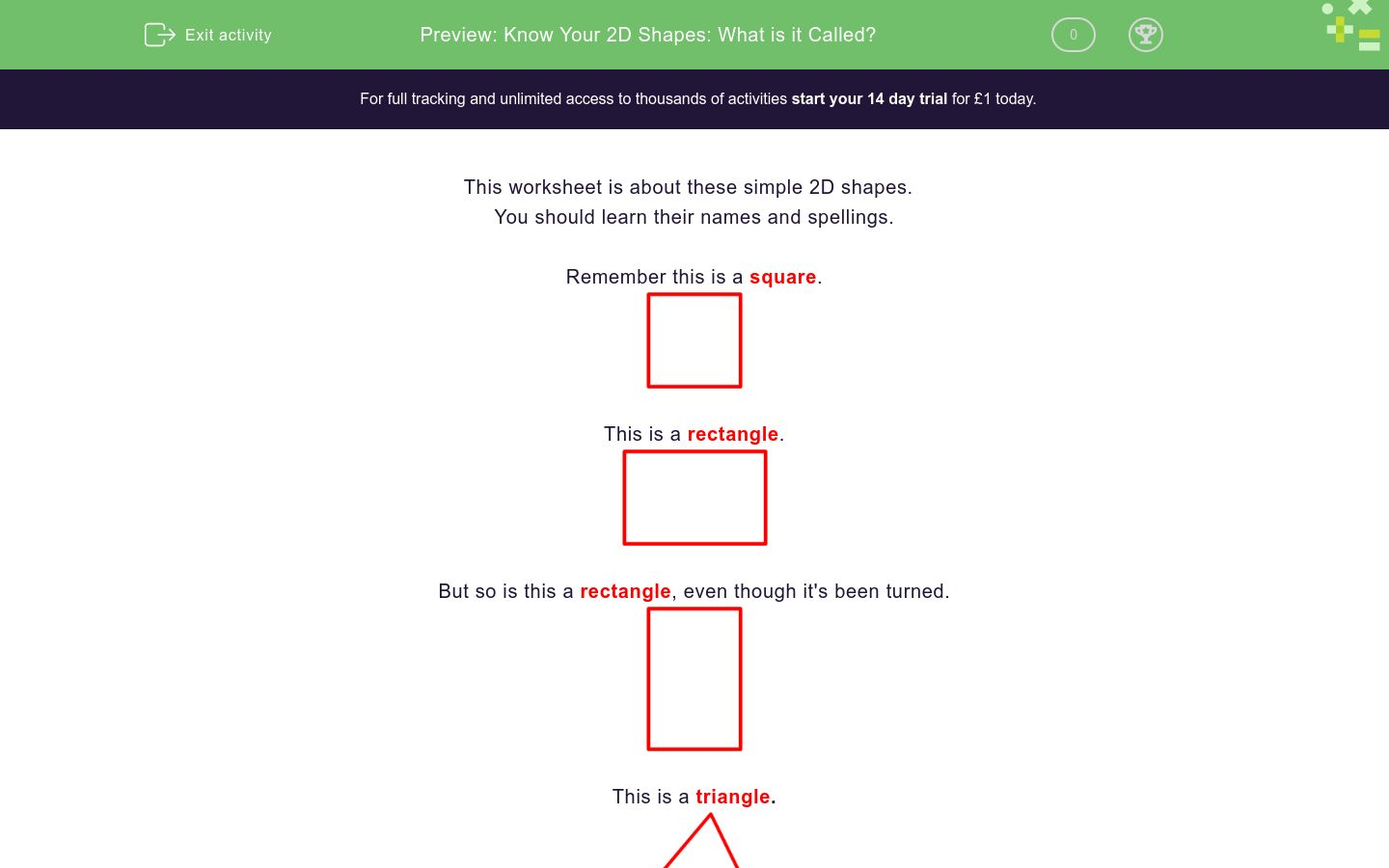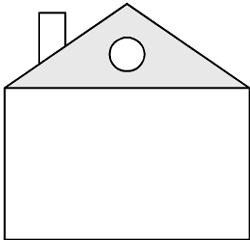# Know Your 2D Shapes: What is it Called?

In this worksheet, students look at pictures drawn with 2D shapes and name them.Key stage:  KS 1

Curriculum topic:   Geometry: Properties of Shapes

Curriculum subtopic:   Recognise 2D and 3D Shapes

Difficulty level:### QUESTION 1 of 10

This worksheet is about these simple 2D shapes.

You should learn their names and spellings.

Remember this is a square.This is a rectangle.But so is this a rectangle, even though it's been turned.This is a triangle.This is a circle.Look at this picture of a house.The wall has 4 sides and opposite sides are equal in length. It has 4 right angles at the corners.

It is a rectangle.

The roof has 3 sides.

It is a triangle.

The window in the roof has 4 equal sides and 4 right angles.

It is a square.

Which shape is the window in the roof?triangle

square

rectangle

circle

Which shape is the roof?triangle

square

rectangle

circle

Which shape is the window in the roof?triangle

square

rectangle

circle

Which shape is the window in the roof?triangle

square

rectangle

circle

Which shape is the window in the roof?triangle

square

rectangle

circle

Which shape are the upstairs windows?triangles

squares

rectangles

circles

Which shape is the downstairs window?triangle

square

rectangle

circle

Which shape is the roof?triangle

square

rectangle

circle

Which shape is the window in the roof?triangle

square

rectangle

circle

Which shape is the door?triangle

square

rectangle

circle

• Question 1

Which shape is the window in the roof?rectangle
EDDIE SAYS
It has 4 sides and 4 right angles.
• Question 2

Which shape is the roof?triangle
EDDIE SAYS
It has 3 sides.
• Question 3

Which shape is the window in the roof?triangle
EDDIE SAYS
It has 3 sides.
• Question 4

Which shape is the window in the roof?circle
EDDIE SAYS
It is round.
• Question 5

Which shape is the window in the roof?square
EDDIE SAYS
It has 4 equal sides and 4 right angles.
• Question 6

Which shape are the upstairs windows?squares
EDDIE SAYS
They have 4 equal sides and 4 right angles.
• Question 7

Which shape is the downstairs window?rectangle
EDDIE SAYS
It has 4 sides and 4 right angles.
• Question 8

Which shape is the roof?triangle
EDDIE SAYS
It has 3 sides.
• Question 9

Which shape is the window in the roof?circle
EDDIE SAYS
It is round.
• Question 10

Which shape is the door?rectangle
EDDIE SAYS
It has 4 sides and 4 right angles.
---- OR ----

Sign up for a £1 trial so you can track and measure your child's progress on this activity.

### What is EdPlace?

We're your National Curriculum aligned online education content provider helping each child succeed in English, maths and science from year 1 to GCSE. With an EdPlace account you’ll be able to track and measure progress, helping each child achieve their best. We build confidence and attainment by personalising each child’s learning at a level that suits them.

Get started